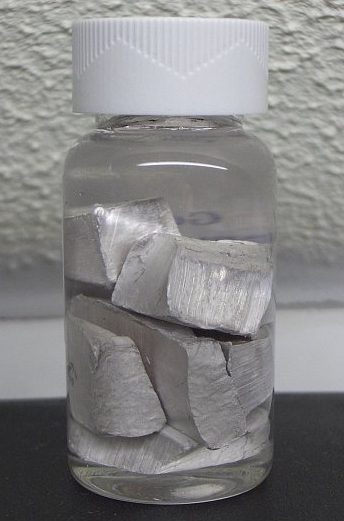Chemistry CIE iGCSE:
Reactivity + Corrosion

Page 1

You will need a copy of the periodic table!

1. Four metals, W, X, Y and Z, were added separately to water and to dilute hydrochloric acid. The observations are given in the table below:

 Metal Reaction with water Reaction with acid W No reaction No reaction X Bubbles slowly Bubbles vigorously Y No reaction Bubbles slowly Z Bubbles slowly Bubbles slowly

What is the order of reactivity of these metals from most to least reactive?

• A.   X , Z , W , Y
• B.   X , Z , Y , W
• C.   X , Y , Z , W
• D.   W , Y , Z , X

2. The following powdered metal and metal oxide mixtures were heated strongly :

 I. Magnesium + zinc oxide II. Copper + iron(III)oxide III. Zinc + copper(II)oxide

Which of the mixtures would react?

• A.   I and II only
• B.   I and III only
• C.   II and III only
• D.   I, II and III

Q3-4:
The reactivity of three different metals, P, Q and R, were investigated by reacting the metals with each other’s sulfate solutions.
The results are given in the table below. A tick indicates a reaction and a cross indicates no reaction.

 Metal P Metal Q Metal R Sulfate of P x x ✔ Sulfate of Q ✔ x ✔ Sulfate of R x x x

3. What is the order of reactivity of these metals from most to least reactive?
• A.  R, P, Q
• B.  Q, P, R
• C.  P, Q, R
• D.  R, Q, P
4. The metal sodium cannot be used in this investigation.The reason that sodium cannot be used is..
• A.   sodium is stored under oil
• B.   sodium does not form a sulfate salt
• C.   sodium will react vigorously with the water in the solution
• D.   sodium is too expensive

Q5-8:
A strip of copper metal was placed in a boiling tube containing silver nitrate solution and left to react.

5. The balanced equation for this reaction is ….
• A. Cu(s) + AgNO3(aq)  CuNO3(aq) + Ag(s)
• B. Cu(s) + 2AgNO3(aq)  Cu(NO3)2(aq) + 2Ag(s)
• C. 2Cu(s) + Ag(NO3)2(aq) 2CuNO3(aq) + Ag(s)
• D. Cu(s) + Ag(NO3)2(aq)  Cu(NO3)2(aq) + Ag(s)

6. What two observations would you expect to make during this reaction?

 Observation 1 Observation 2 A bubbles colourless solution turns pale blue B bubbles blue solution turns colourless C Grey coating on the copper colourless solution turns pale blue D Grey coating on the copper blue solution turns colourless

7. In this reaction explain whether the copper is oxidized or reduced.

 Copper is.. Because copper.. A oxidised Lost electrons B oxidised Gained electrons C reduced Lost electrons D reduced Gained electrons
8. This reactions shows us that..
• A.   copper is more reactive than silver
• B.   silver is more reactive than copper
• C.   copper is more reactive than silver nitrate
• D.   silver nitrate is more reactive than copper
9. Aluminium metal is used for food containers because it does not corrode but it is actually more reactive than iron.

What is the reason for the apparent unreactivity of aluminium?

• A.   Aluminium does not react with oxygen
• B.   Aluminium forms an oxide layer which adheres to the metal
• C.   Aluminium forms positive ions less easily than iron
• D.   Aluminium is alloyed to prevent it from corroding
10. A student used zinc and dilute sulfuric acid in the following experiment to collect a sample of hydrogen gas:Which two other metals could the student have safely used to produce a sample of hydrogen?

• A.   copper and silver
• B.   potassium and magnesium
• C.   sodium and copper
• D.   magnesium and iron